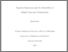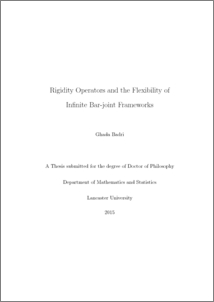# Rigidity operators and the flexibility of infinite bar-joint frameworks

Badri, Ghada (2015) Rigidity operators and the flexibility of infinite bar-joint frameworks. PhD thesis, UNSPECIFIED.Preview

## Abstract

In this thesis we bring together various techniques from functional analysis and operator theory to develop the linear infinitesimal theory of crystal frameworks in the Euclidean space Rd. In this mathematical theory we obtain sufficient conditions for the boundedness of the rigidity matrix R(G), viewed as a Hilbert space operator, for certain infinite tree frameworks. Also, we provide an analysis of the vector subspace of strictly periodic flexes implied by the translational symmetry of crystal frameworks and we prove a relation in which the space of supercell n-fold periodic flexes can be written as a direct sum of the relevant vector subspaces of phase periodic flexes. A main result in the thesis is the development of the almost periodic rigidity theory for crystal frameworks in Rd. We prove that a crystal framework is almost periodically infinitesimally rigid if and only if it is periodically in-finitesimally rigid and the corresponding RUM spectrum is the minimal set {(1, 1, . . . , 1)}. Finally, we conclude the thesis by defining and identifying crystal flex bases for the real vector space of all infinitesimal flexes of a crystal framework C. In particular, we determine a crystal flex basis for the crystal framework COct formed by pairwise vertex connected regular octahedra. This bar-joint framework (for the mineral perovskite) features in early papers on the investigation of rigid unit modes in material crystals.

Item Type:
Thesis (PhD)
Departments:
ID Code:
74367
Deposited By:
Deposited On:
18 Jun 2015 06:18
Refereed?:
No
Published?:
Published